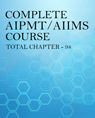Click to Chat

1800-1023-196

+91-120-4616500

CART 0

• 0

MY CART (5)

Use Coupon: CART20 and get 20% off on all online Study Material

ITEM
DETAILS
MRP
DISCOUNT
FINAL PRICE
Total Price: Rs.

There are no items in this cart.
Continue Shopping

Cp - Cv value of real gases would be more or less than that of ideal gas....see img.....
9 months ago

If you follow the proof, you can see that the ‘nb’ term makes no difference to either the specific heat at constant pressure or at constant volume.At constant pressure the amount of heat, Q, needed to produce a temperature change ΔT at constant pressure for n moles of ANY gas is:Q = nCpΔTWhere Cp is the molar specific heat at constant pressure.The energy supplied, Q, can be considered as increasing in internal energy (ΔU) and doing work at constant pressure, PΔV. SoΔU + PΔV = nCpΔTΔU = nCpΔT - PΔV (equation 1)_____________________Consider an ideal gas obeying PV=nRT, where P is constant.If V increases by ΔV and T increases by ΔTP(V+ΔV) = =nR(T+ΔTPV+ PΔV = =nRT+ nRΔTSubtracting ‘PV = nRT’ gives:PΔV = nRΔTFrom equation 1ΔU = n.Cp. ΔT - nR ΔT= nΔT(Cp - R)ΔU/ΔT = n(Cp - R) (equation 2)______________________Now repeat the above calculation for a real gas obeying P(V-nb)=nRT. where P is constant.PV – Pnb = nRTIf V increases by ΔV and T increase by ΔTP(V+ΔV + nb) =nR(T+ΔT)PV+ PΔV + Pnb =nRT+ nRΔT)Subtracting ‘PV – Pnb = nRT’ gives:PΔV = nRΔTNote that the ‘nb’ term has disappeared and we are left with the same formula as for an ideal gas.The same calculation used above for an deal gas then applies soΔU/ΔT = n(Cp - R) (same equation 2 as above)_____________________At constant volume, a gas does no work. So only internal energy (U) changes.If Cv is the molar specific heat at constant volumeQ = ΔU = nCvΔTΔU/ ΔT = nCv.This is true for the ideal gas and for the real gas.From equation 2nCv. = n(Cp - R)Cp – Cv – RSo Cp – Cv = R is true for both the ideal and the real gas
9 months ago
Think You Can Provide A Better Answer ?
Answer & Earn Cool Goodies

Other Related Questions on AIIMS MBBS

View all Questions »Course Features

• 731 Video Lectures
• Revision Notes
• Previous Year Papers
• Mind Map
• Study Planner
• NCERT Solutions
• Discussion Forum
• Test paper with Video SolutionCourse Features

• 728 Video Lectures
• Revision Notes
• Test paper with Video Solution
• Mind Map
• Study Planner
• NCERT Solutions
• Discussion Forum
• Previous Year Exam Questions